Next: What is Up: Special Numbers and Functions Previous: How to compute digits

# Euler's formula:

The definition and domain of exponentiation has been changed several times. The original operation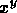was only defined when y was a positive integer. The domain of the operation of exponentation has been extended, not so much because the original definition made sense in the extended domain, but because there were (almost) unique ways to extend exponentation which preserved many of what seemed to be the ``important" properties of the original operation. So in part, these definitions are only convention, motivated by reasons of aesthetics and utility.

The original definition of exponentiation is, of course, that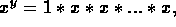where 1 is multiplied by x, y times. This is only a reasonable definition for y=1, 2, 3, ... (It could be argued that it is reasonable when y=0, but that issue is taken up in a different part of the FAQ). This operation has a number of properties, including

1.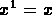2. For any x, n, m,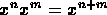.
3. If x is positive, then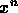is positive.

Now, we can try to see how far we can extend the domain of exponentiation so that the above properties (and others) still hold. This naturally leads to defining the operationon the domain x positive real; y rational, by setting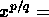the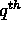root of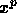. This operation agrees with the original definition of exponentiation on their common domain, and also satisfies (1), (2) and (3). In fact, it is the unique operation on this domain that does so. This operation also has some other properties:

4. If x>1, thenis an increasing function of y.
5. If 0<x<1, thenis a decreasing function of y.

Again, we can again see how far we can extend the domain of exponentiation while still preserving properties (1)-(5). This leads naturally to the following definition ofon the domain x positive real; y real:

If x>1,is defined to be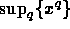, where q runs over a ll rationals less than or equal to y.

If x<1,is defined to be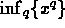, where q runs over a ll rationals bigger than or equal to y.

If x=1,is defined to be 1.

Again, this operation satisfies (1)-(5), and is in fact the only operation on this domain to do so.

The next extension is somewhat more complicated. As can be proved using the methods of calculus or combinatorics, if we define e to be the number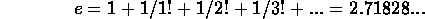it turns out that for every real number x,

6.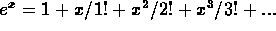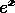is also denoted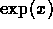. (This series always converges regardless of the value of x).

One can also define an operation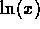on the positive reals, which is the inverse of the operation of exponentiation by e. In other words,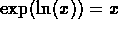for all positive x. Moreover,

7. If x is positive, then=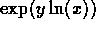. Because of this, the natural extension of exponentiation to complex exponents, seems to be to define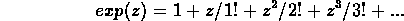for all complex z (not just the reals, as before), and to define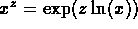when x is a positive real and z is complex.

This is the only operationon the domain x positive real, y complex which satisfies all of (1)-(7). Because of this and other reasons, it is accepted as the modern definition of exponentiation.

From the identities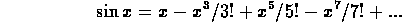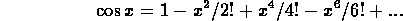which are the Taylor series expansion of the trigonometric sine and cosine functions respectively. From this, one sees that, for any real x,

8.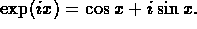Thus, we get Euler's famous formula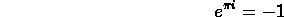and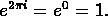One can also obtain the classical addition formulae for sine and cosine from (8) and (1).

All of the above extensions have been restricted to a positive real for the base. When the base x is not a positive real, it is not as clear-cut how to extend the definition of exponentiation. For example,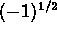could well be i or -i,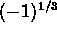could be -1,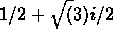, or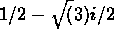, and so on. Some values of x and y give infinitely many candidates for, all equally plausible. And of course x=0 has its own special problems. These problems can all be traced to the fact that the exp function is not injective on the complex plane, so that ln is not well defined outside the real line. There are ways around these difficulties (defining branches of the logarithm, for example), but we shall not go into this here.

The operation of exponentiation has also been extended to other systems like matrices and operators. The key is to define an exponential function by (6) and work from there. [Some reference on operator calculus and/or advanced linear algebra?]

References

Complex Analysis. Ahlfors, Lars V. McGraw-Hill, 1953.Next: What is Up: Special Numbers and Functions Previous: How to compute digits

Alex Lopez-Ortiz
Mon Feb 23 16:26:48 EST 1998